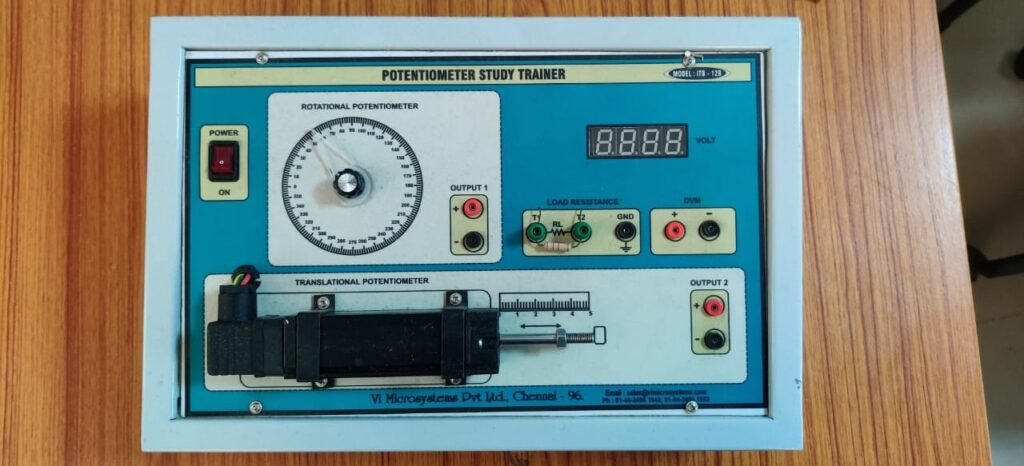# Topic 5 Resistive Input Transducersresistive transducer is a device that senses a change to cause a change in resistance.  Transducers do NOT generate electricity.  Examples include:

 Device Action Where used Light Dependent Resistor Resistance falls with increasing light level Light operated switches Thermistor Resistance falls with increased temperature Electronic thermometers Strain gauge Resistance changes with force Sensor in an electronic balance Moisture detector Resistance falls when wet Damp meter

These are called passive devices.  (Active transducers do generate electricity from other energy sources, or have a power supply.)

Now have a go at Question 1 and Question 2

# Light Dependent Resistors

The light dependent resistor consists of a length of material (cadmium sulphide) whose resistance changes according to the light level.    Therefore the brighter the light, the lower the resistance.

We can show the way the resistance varies with light level as a graph:

The first graph shows us the variation using a linear scale.   The graph on the right shows the plot as a logarithmic plot, which comes up as a straight line.  Logarithmic plots are useful for compressing scales.

LDRs are used for:

• Smoke detection
•  Automatic lighting
• Counting
• Alarm systems.

Resistive components can get hot when excessive current is flowing through them, and this can impair their function, or damage them.  This can be prevented by connecting a current limiting resistor in series, as shown in the picture below.

 Worked Example:At a certain light level, a light dependent resistor has a resistance of 100 ohms.  It can only handle a current of 10 milliamps before it risks heating up.  If the LDR is connected to a 20 V supply, what value resistor should we place in series? Continue

Now try one for yourself with Question 3

Thermistors

The most common type of thermistor that we use has a resistance that falls as the temperature rises.  It is referred to as a negative temperature coefficient device.  A positive temperature coefficient device has a resistance that increases with temperature.

The graph of resistance against temperature is like this.

The resistance on this graph is on a logarithmic scale, as there is a large range of values.

The LDR is most commonly used in a potential divider circuit.

Potential Divider

• Although it is simple, the potential divider is a very useful circuit.  In its simplest form it is two resistors in series with an input voltage Vs across the ends.
• An output voltage Vout is obtained from a junction between the two resistors.

The potential divider circuit looks like this:

You need to learn this equation.  It is very useful.

This result can be thought of as the output voltage being the same fraction of the input voltage as R2 is the fraction of the total resistance.  Look at this circuit for the next example: